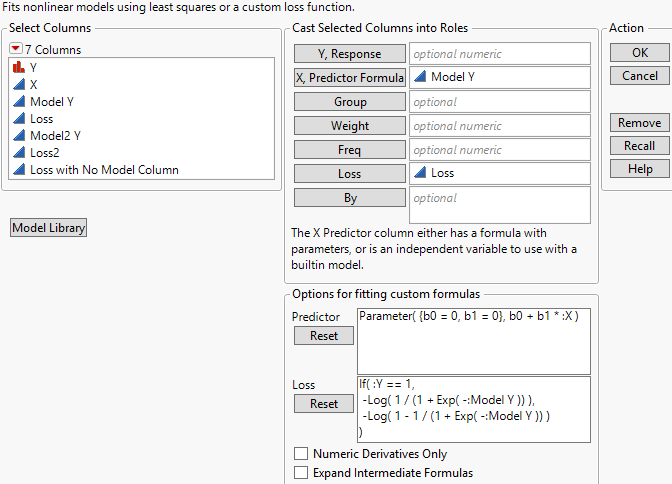Publication date: 03/23/2021

# Launch the Nonlinear Platform

To launch the Nonlinear platform, select Analyze > Specialized Modeling > Nonlinear.

Figure 15.4 Nonlinear Platform Launch WindowFor more information about the options in the Select Columns red triangle menu, see Column Filter Menu in Using JMP.

The Nonlinear platform launch window has the following features:

Y, Response

Select the Y variable.

X, Predictor Formula

Select either the X variable or a column containing the model formula with parameters.

Note: If you select a column that does not contain a model formula with parameters and you also do not specify a custom formula, the Fit Curve platform is launched instead.

Note: If you select a formula column that contains a parameter list item that does not have an assignment, JMP automatically sets that parameter to zero and displays a message in the log.

Group

Specify a grouping variable. The fitted model has separate parameters for each level of the grouping variable. This enables you to compare fitted models and estimated parameters across the levels of the grouping variable.

Note: If the formula does not have separate parameters for each group level, the Group variable is ignored by JMP.

Weight

Specify a variable containing the weights of observations.

Freq

Specify a variable representing the frequency of an observation.

Loss

Specify a formula column giving a loss function.

By

Specify a variable to perform a separate analysis for every level of the variable.

Model Library

Launches the Model Library tool, which helps you choose initial values to create a formula column. See Create a Formula Using the Model Library.

Options for fitting custom formulas

If you specify a column in the X, Predictor Formula role that does not contain a model formula with parameters, you can use this option to create a custom formula. In the Predictor field, specify parameters, parameter values, and a custom formula that uses the selected X variable. Then, click the Reset button and launch the platform.

Numeric Derivatives Only

Uses numeric derivatives only. This option is useful when you have a model for which it is too messy to take analytic derivatives. It can also be valuable in obtaining convergence in tough cases. This option is used only when a formula column is provided in the X, Predictor Formula role.

Expand Intermediate Formulas

Tells JMP that if an ingredient column to the model is a column that itself has a formula, to substitute the inner formula, as long as it refers to other columns. To prevent an ingredient column from expanding, use the Other column property with a name of “Expand Formula” and a value of 0. This option is used only when a formula column is provided in the X, Predictor Formula role.

Want more information? Have questions? Get answers in the JMP User Community (community.jmp.com).